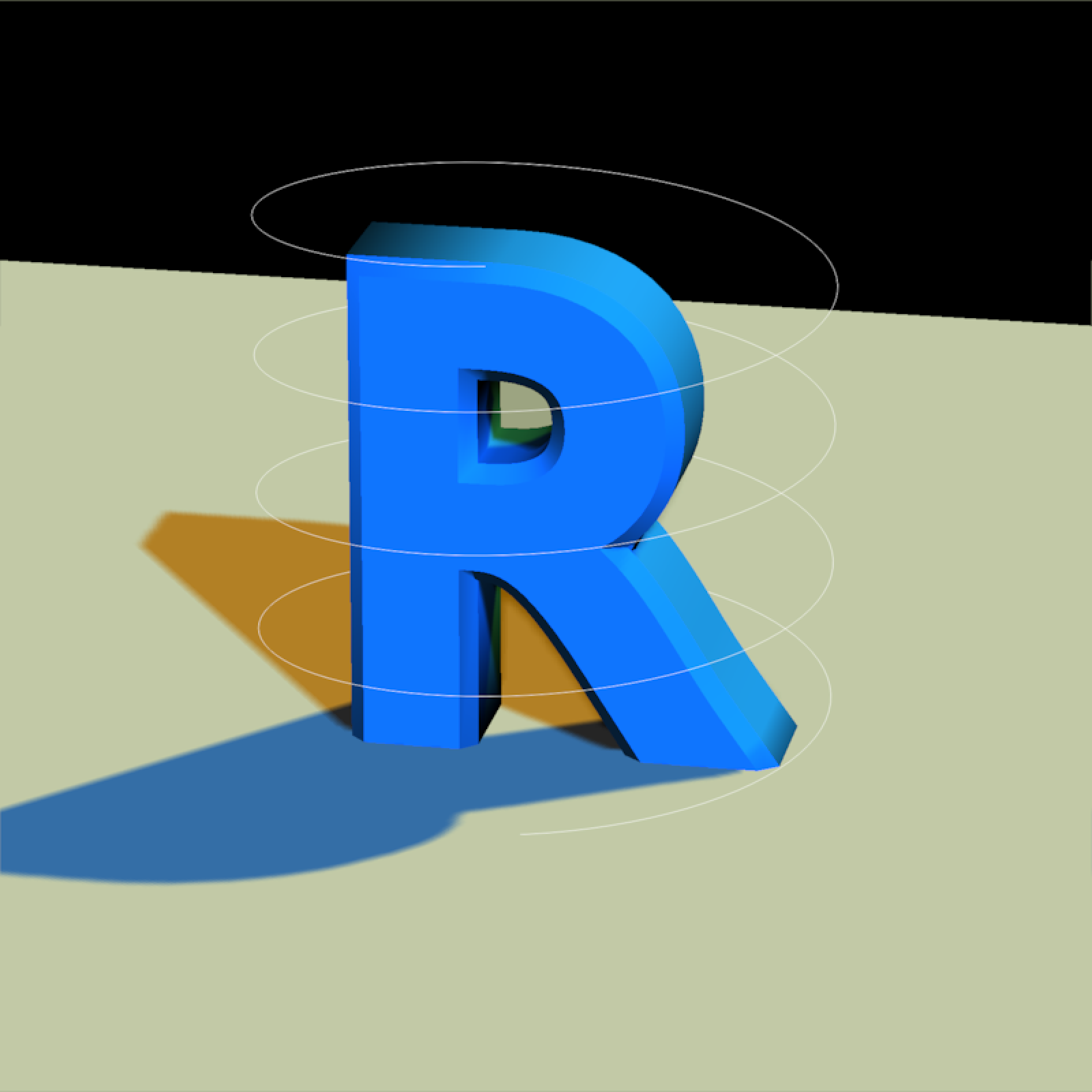Render a 3D scene with meshes, lights, and lines using a software rasterizer.

rasterize_scene(
scene,
filename = NA,
width = 800,
height = 800,
line_info = NULL,
alpha_line = 1,
parallel = TRUE,
fov = 20,
lookfrom = c(0, 0, 10),
lookat = NULL,
camera_up = c(0, 1, 0),
fsaa = 2,
light_info = directional_light(),
color = "red",
type = "diffuse",
background = "black",
tangent_space_normals = TRUE,
ssao = FALSE,
ssao_intensity = 10,
tonemap = "none",
debug = "none",
near_plane = 0.1,
far_plane = 100,
block_size = 4,
shape = NULL,
line_offset = 1e-05,
ortho_dimensions = c(1, 1),
bloom = FALSE,
antialias_lines = TRUE,
environment_map = "",
background_sharpness = 1,
verbose = FALSE,
vertex_transform = NULL,
validate_scene = TRUE
)

## Arguments

scene

The scene object.

filename

Default NULL. Filename to save the image. If NULL, the image will be plotted.

width

Default 400. Width of the rendered image.

height

Default 400. Width of the rendered image.

line_info

Default NULL. Matrix of line segments to add to the scene. Number of rows must be a multiple of 2.

alpha_line

Default 1. Line transparency.

parallel

Default TRUE. Whether to use parallel processing.

fov

Default 20. Width of the rendered image.

lookfrom

Default c(0,0,10). Camera location.

lookat

Default NULL. Camera focal position, defaults to the center of the model.

camera_up

Default c(0,1,0). Camera up vector.

fsaa

Default 2. Full screen anti-aliasing multiplier. Must be positive integer, higher numbers will improve anti-aliasing quality but will vastly increase memory usage.

light_info

Default directional_light(). Description of scene lights, generated with the point_light() and directional_light() functions.

color

Default darkred. Color of model if no material file present (or for faces using the default material).

type

Default diffuse. Shader type. Other options: vertex (Gouraud shading), phong, and color (no lighting).

background

Default white. Background color.

tangent_space_normals

Default TRUE.

Default FALSE.

Default 0.005.

Default 0.5.

Default NULL.

ssao

Default FALSE. Whether to add screen-space ambient occlusion (SSAO) to the render.

ssao_intensity

Default 10. Intensity of the shadow map.

Default 0.1. Radius to use when calculating the SSAO term.

tonemap

Default "none".

debug

Default "none".

near_plane

Default 0.1.

far_plane

Default 100.

Default "default".

block_size

Default 4.

shape

Default NULL. The shape to render in the OBJ mesh.

line_offset

Default 0.0001. Amount to offset lines towards camera to prevent z-fighting.

ortho_dimensions

Default c(1,1). Width and height of the orthographic camera. Will only be used if fov = 0.

bloom

Default FALSE. Whether to apply bloom to the image. If TRUE, this performs a convolution of the HDR image of the scene with a sharp, long-tailed exponential kernel, which does not visibly affect dimly pixels, but does result in emitters light slightly bleeding into adjacent pixels.

antialias_lines

Default TRUE. Whether to anti-alias lines in the scene.

environment_map

Default "". Image file to use as a texture for all reflective and refractive materials in the scene, along with the background.

background_sharpness

Default 1.0. A number greater than zero but less than one indicating the sharpness of the background image.

verbose

Default FALSE. Prints out timing information.

vertex_transform

Default NULL. A function that transforms the vertex locations, based on their location. Function should takes a length-3 numeric vector and returns another length-3 numeric vector as the output.

validate_scene

Default TRUE. Whether to validate the scene input.

## Value

Rasterized image.

## Examples

if(rayvertex:::run_documentation()) {
#Let's load the cube OBJ file included with the package

rasterize_scene(cube_mesh(),lookfrom=c(2,4,10),
light_info = directional_light(direction=c(0.5,1,0.7)))
}
#> Setting lookat to: c(0.00, 0.00, 0.00)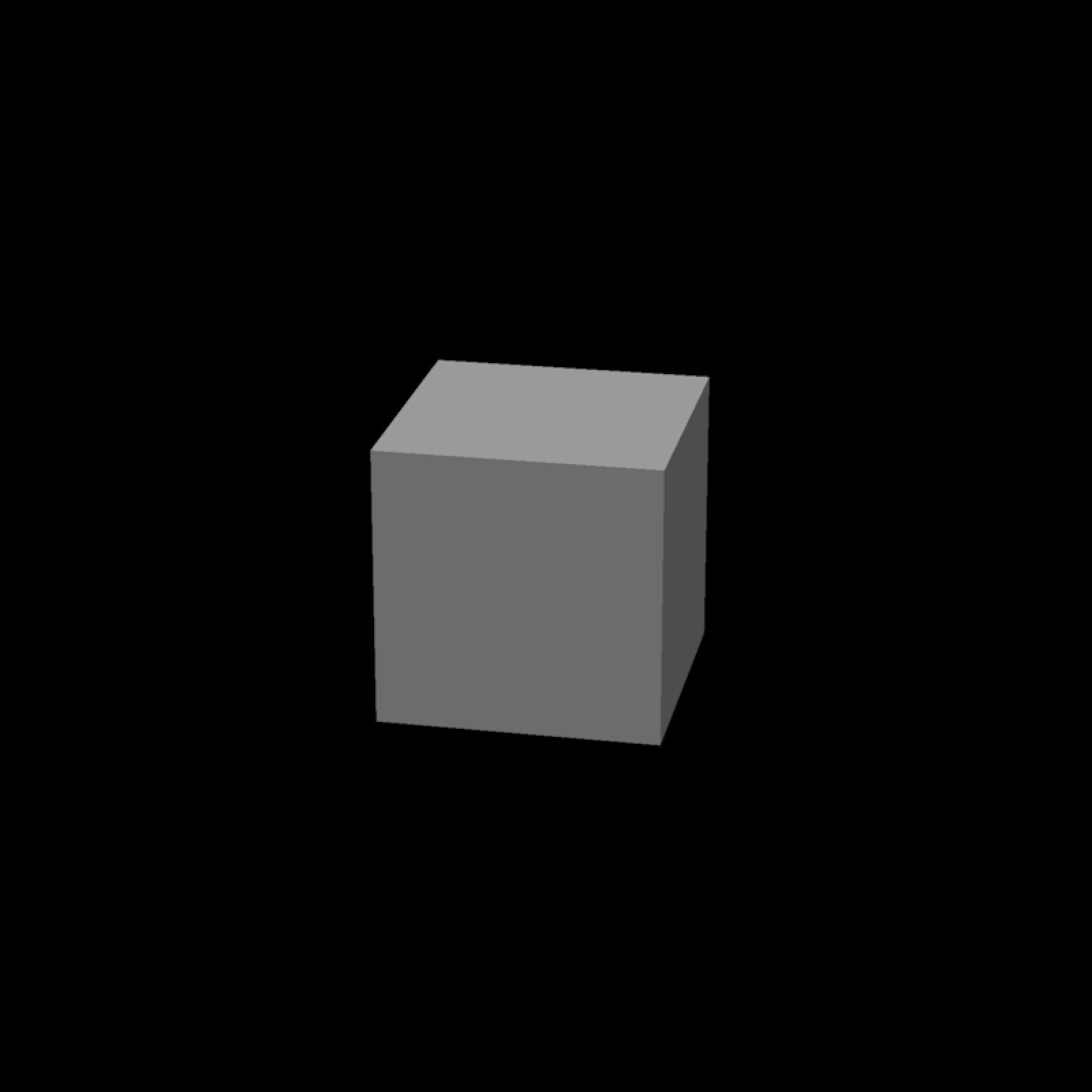if(rayvertex:::run_documentation()) {
#Flatten the cube, translate downwards, and set to grey
base_model = cube_mesh() |>
scale_mesh(scale=c(5,0.2,5)) |>
translate_mesh(c(0,-0.1,0)) |>
set_material(diffuse="grey80")

rasterize_scene(base_model, lookfrom=c(2,4,10),
light_info = directional_light(direction=c(0.5,1,0.7)))
}
#> Setting lookat to: c(0.00, -0.10, 0.00)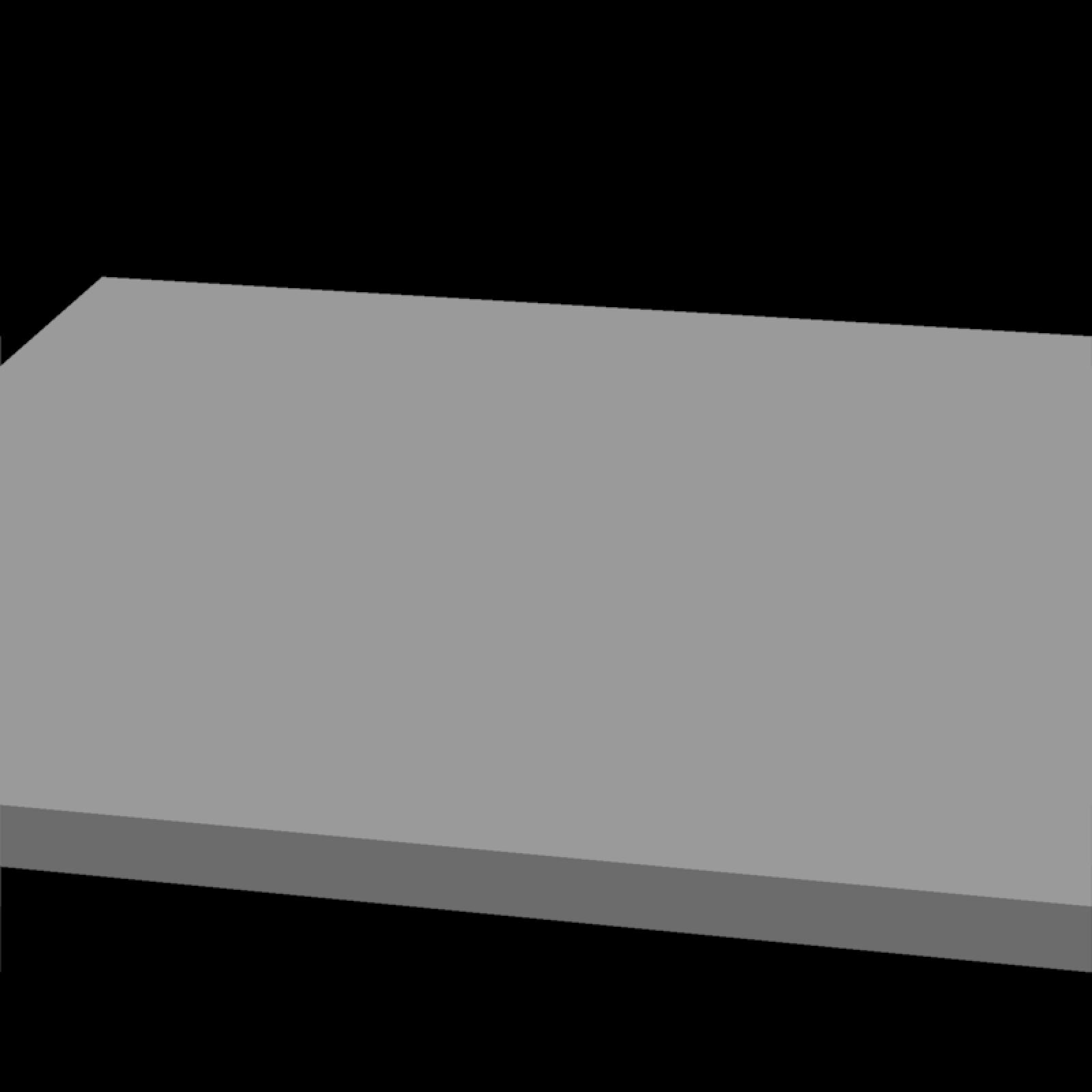if(rayvertex:::run_documentation()) {
#load the R OBJ file, scale it down, color it blue, and add it to the grey base
r_model = obj_mesh(r_obj()) |>
scale_mesh(scale=0.5) |>
set_material(diffuse="dodgerblue") |>

rasterize_scene(r_model, lookfrom=c(2,4,10),
light_info = directional_light(direction=c(0.5,1,0.7)))
}
#> Setting lookat to: c(0.00, 0.34, 0.00)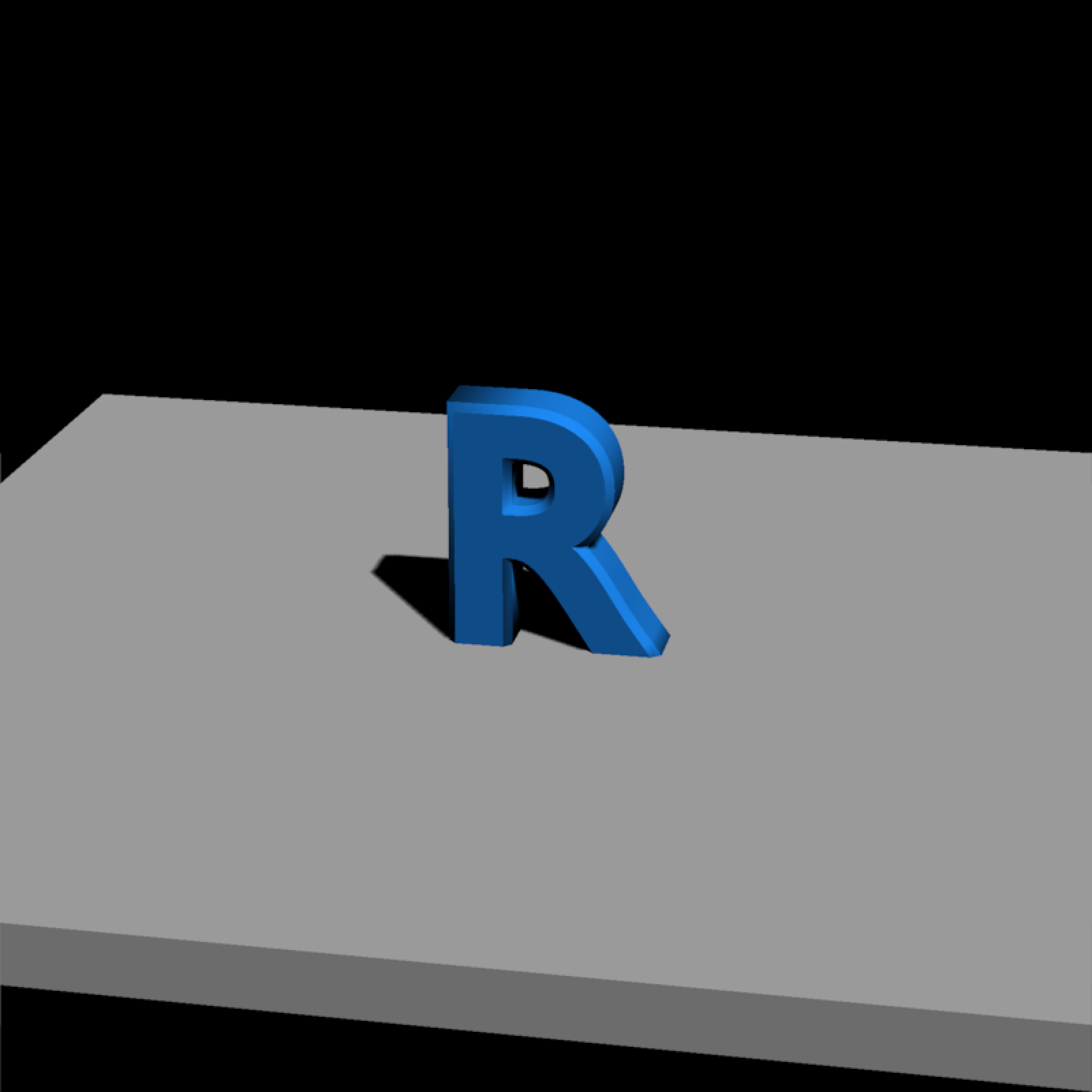if(rayvertex:::run_documentation()) {
#Zoom in and reduce the shadow mapping intensity
light_info = directional_light(direction=c(0.5,1,0.7)))
}
#> Setting lookat to: c(0.00, 0.34, 0.00)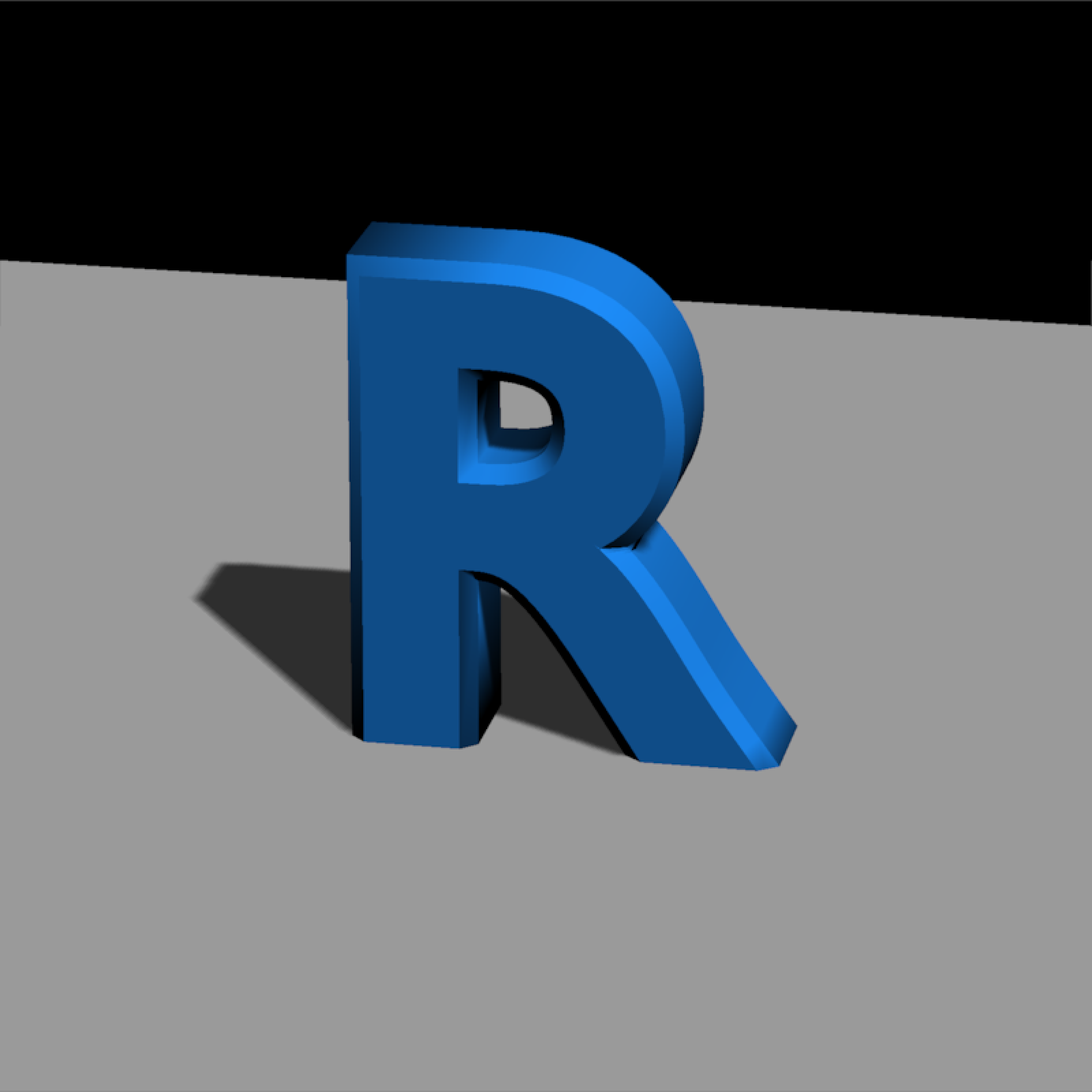if(rayvertex:::run_documentation()) {
#Include the resolution (4x) of the shadow map for less pixellation around the edges
#Also decrease the shadow_map_bias slightly to remove the "peter panning" floating shadow effect
rasterize_scene(r_model, lookfrom=c(2,4,10), fov=10,
light_info = directional_light(direction=c(0.5,1,0.7)))
}
#> Setting lookat to: c(0.00, 0.34, 0.00)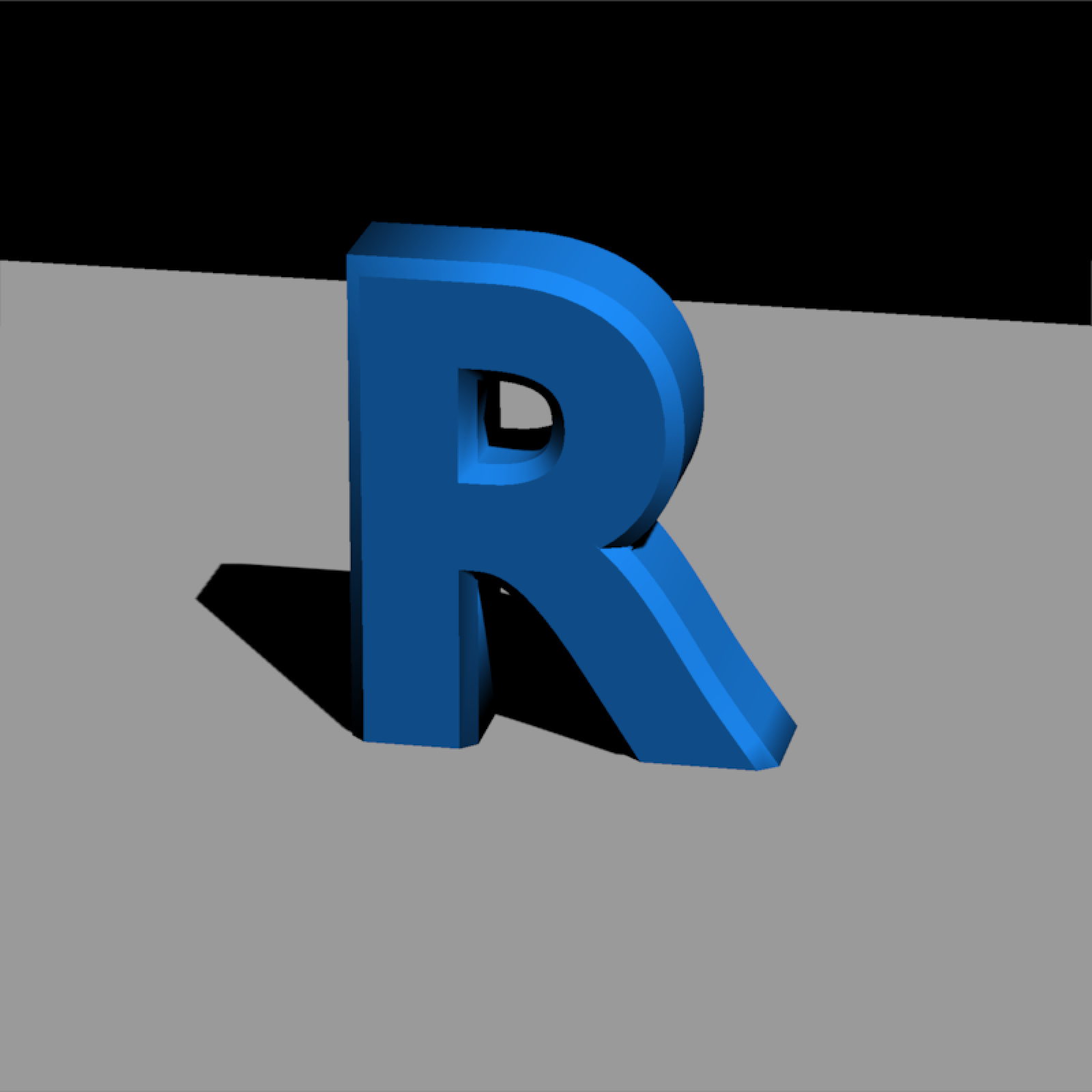if(rayvertex:::run_documentation()) {
#Add some more directional lights and change their color
lights = directional_light(c(0.7,1.1,-0.9),color = "orange",intensity = 1) |>
add_light(directional_light(c(0.7,1,1),color = "dodgerblue",intensity = 1)) |>
rasterize_scene(r_model, lookfrom=c(2,4,10), fov=10,
light_info = lights)
}
#> Setting lookat to: c(0.00, 0.34, 0.00)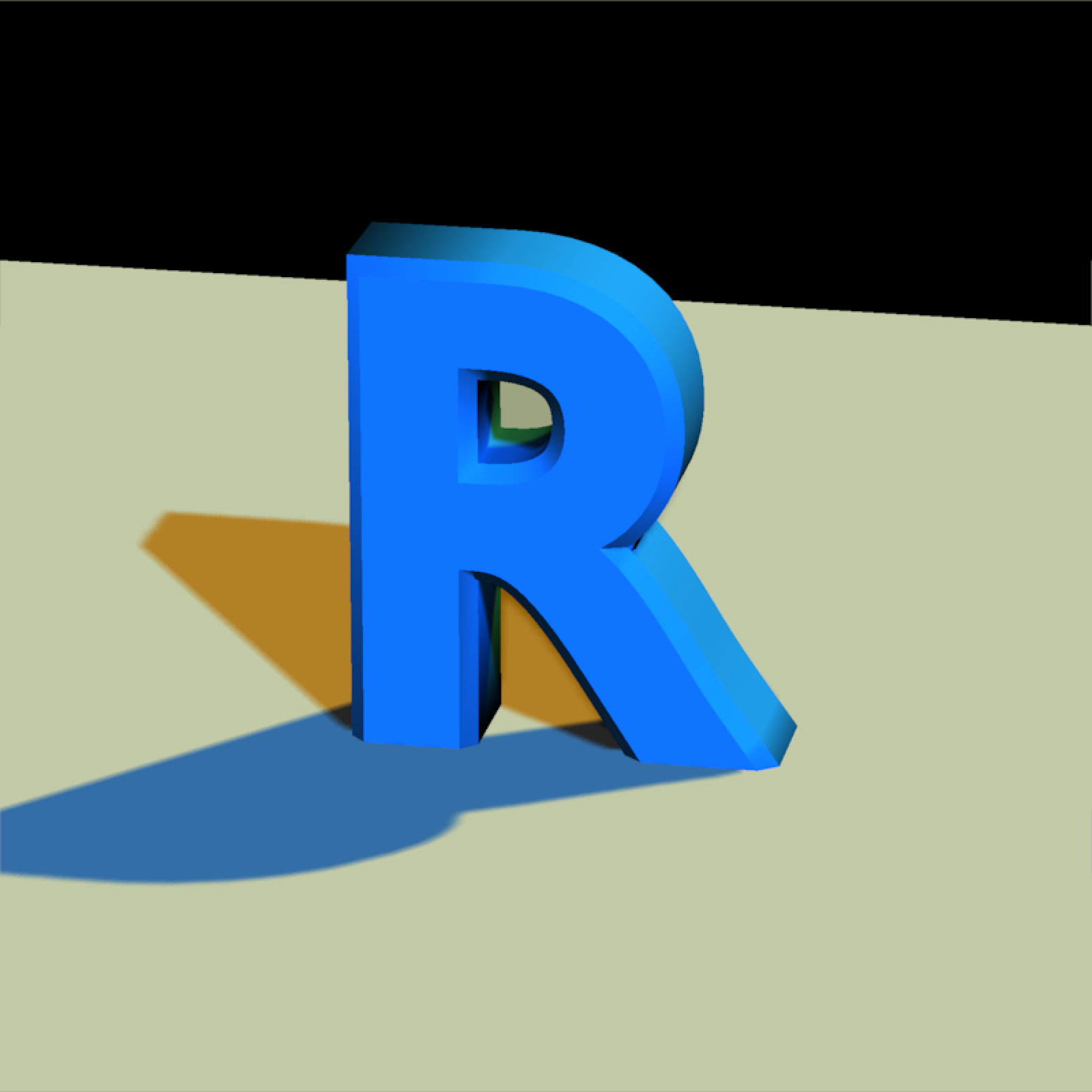if(rayvertex:::run_documentation()) {
lights_p = lights |>
rasterize_scene(r_model, lookfrom=c(2,4,10), fov=10,
light_info = lights_p)
}
#> Setting lookat to: c(0.00, 0.34, 0.00)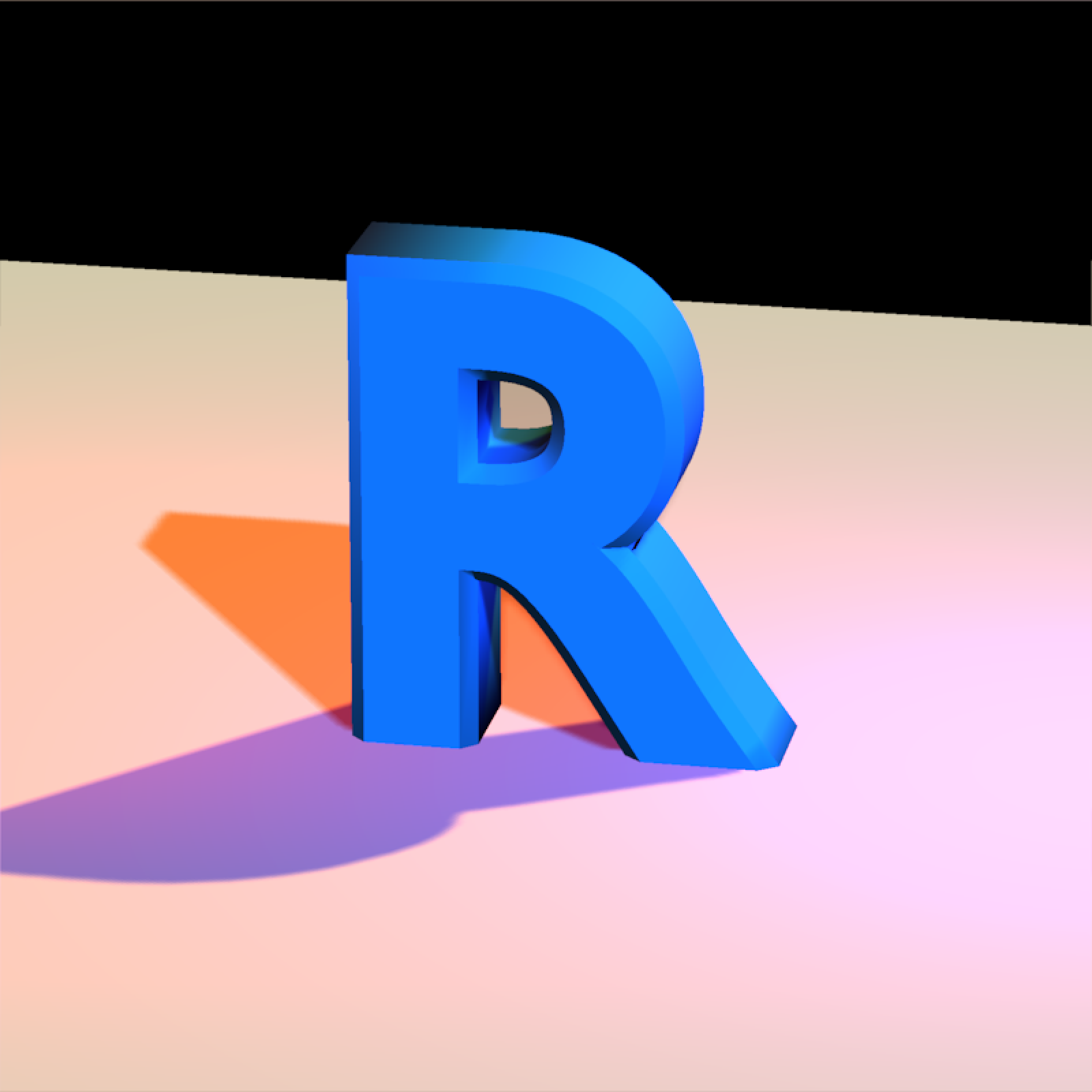if(rayvertex:::run_documentation()) {
#change the camera position
rasterize_scene(r_model, lookfrom=c(-2,2,-10), fov=10,
light_info = lights_p)
}
#> Setting lookat to: c(0.00, 0.34, 0.00)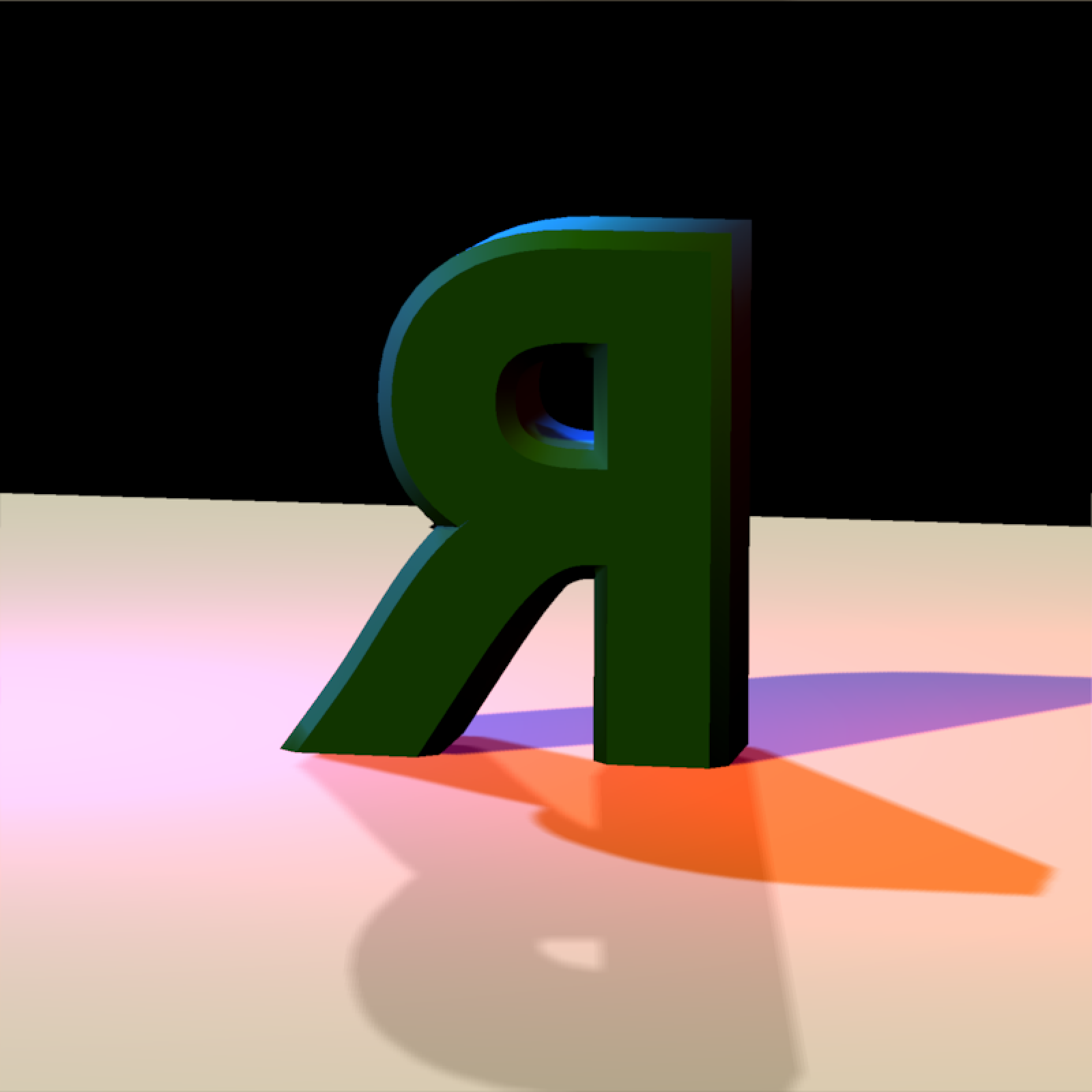if(rayvertex:::run_documentation()) {

#Add a spiral of lines around the model by generating a matrix of line segments
t = seq(0,8*pi,length.out=361)
line_mat = matrix(nrow=0,ncol=9)

for(i in 1:360) {
#> Setting lookat to: c(0.00, 0.34, 0.00)